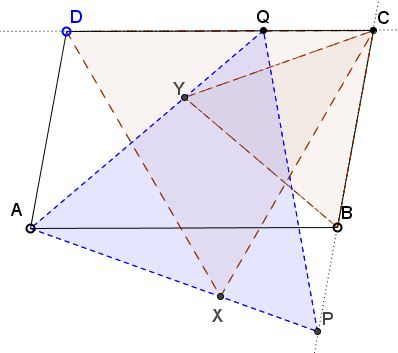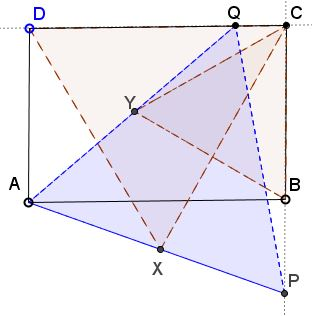Equilateral Triangles On Sides of a Parallelogram IIWhat Is It About? A Mathematical Droodle

ExplanationThe applet below provides an illustration to a generalization of a problem that appeared in P. Yiu's article Elegant Geometric Constructions:

Given a rectangle $ABCD,$ to find points $P$ and $Q$ on $BC$ and $CD,$ respectively, such that $\Delta APQ$ is equilateral, first construct equilateral triangles $CDX$ and $BCY,$ with $X$ and $Y$ inside the rectangle. Then extend $AX$ to intersect $BC$ at $P$ and $AY$ to intersect $CD$ at $Q.$ The triangle $APQ$ is equilateral.

As can be seen from the applet, the construction works for any parallelogram, the main requirement being that triangles $BCY$ and $CDX$ have the same orientation.P. Yiu's construction is elegant, but its validation by calculating the lengths of several straight line segments is not. Here is a really elegant substitute.

In the original case where $ABCD$ is a rectangle, $\Delta AXY$ is equilateral.(This is because of a property of equilateral triangles constructed on the sides of a parallelogram.) By considering the trapezoid $ABCQ,$ we see that $Y$ is the midpoint of $AQ.$ Similarly, in the trapezoid $ADCP,$ $X$ is the midpoint of $AP.$ Hence, $\Delta APQ$ is obtained by homothety with coefficient 2 from $\Delta AXY.$ Since the latter is equilateral, so is the former.

In general, as professor W. McWorter has observed, $\Delta AXY$ is the affine sum of $\Delta BCY$ and $\Delta DXC;$ it is therefore similar to the two. He also remarked that the similarity of triangles $AXY$ and $APQ$ follows more directly from the proportion $AY : AQ = AX : AP$ which makes $XY\parallel PQ.$

An anonymous visitor has remarked that, in a general case, triangles $ABY$ and $XCY$ are congruent, pretty much by construction - only $\angle ABY = \angle XCY$ is needed for SAS - to conclude that $AY = XY$. To prove $\angle ABY = \angle XCY$ one ought to consider two cases where the acute angle in $ABCD$ is less or greater than $60^{\circ}$ but, otherwise, the derivation is immediate. As a consequence, $\angle AYB = \angle CYX.$ Therefore,

$\angle AYX = \angle BYC + \angle AYB - \angle CYX = \angle BYC = π/3.$

References

1. P. Yiu, Elegant Geometric Constructions, Forum Geometricorum, 5 (2005), pp. 75-96• A problem with equilateral triangles: an interactive illustration
• Six Incircles in Equilateral Triangle
• Equilateral Triangle, Straight Line and Tangent Circles
• Equilateral Triangles on Diagonals of Antiequilic Quadrilateral
• Equilateral Triangles On Sides of a Parallelogram
• Equilateral Triangles on Sides of a Parallelogram III
• Triangle Classification
• Equilateral Triangles and Incircles in a Square
• Equal Areas in Equilateral Triangle
• A Circle Rolling in an Equilateral Triangle
• Viviani's Theorem
• Regular Polygons in a Triangular Grid
• Common Centroids Lead to Equilateral Triangle
•